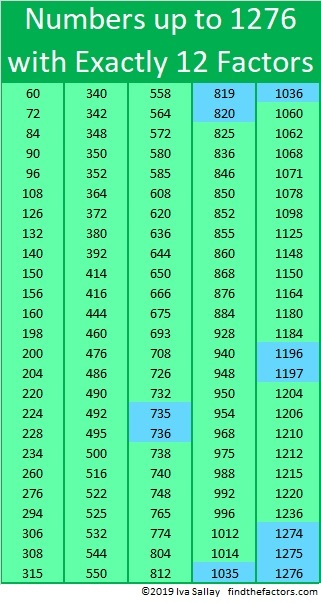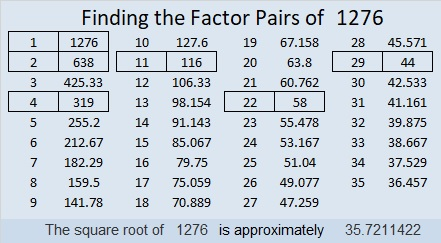# 1276 is the Third Number in a Row with Exactly 12 Factors

1276 is the 125th number to have exactly 12 factors. I’ve made a list of those numbers in the graphic below. Two consecutive numbers appearing on the list have only happened three times before. Those numbers are highlighted in blue. 1276 is special because with it, for the first time THREE consecutive numbers appear on the list!Look at the prime factorizations of those three consecutive numbers:
1274 = 2·7²·13
1275 = 3·5²·17
1276 = 2²·11·29

How are they the same? Can you figure out a reason why they all have exactly 12 factors?

By the way, prime number 1277 spoiled the streak especially since 1278 = 2·3²·71 and also has 12 factors!

If you came up with a rule, I think you should know that not all numbers with 12 factors will follow that same rule. For example,
2³·3² = 72 and has 12 factors because 4·3=12.
2⁵·3 = 96 and has 12 factors because 6·2 = 12.

Now I’ll tell you some more facts about the number 1276:

• 1276 is a composite number.
• Prime factorization: 1276 = 2 × 2 × 11 × 29, which can be written 1276 = 2² × 11 × 29
• The exponents in the prime factorization are 2, 1, and 1. Adding one to each and multiplying we get (2 + 1)(1 + 1)(1 + 1) = 3 × 2 × 2 = 12. Therefore 1276 has exactly 12 factors.
• Factors of 1276: 1, 2, 4, 11, 22, 29, 44, 58, 116, 319, 638, 1276
• Factor pairs: 1276 = 1 × 1276, 2 × 638, 4 × 319, 11 × 116 22 × 58, or 29 × 44
• Taking the factor pair with the largest square number factor, we get √1276 = (√4)(√319) = 2√319 ≈ 35.721141276 is the sum of 12 consecutive prime numbers:
79 + 83 + 89 + 97 + 101 + 103 + 107 + 109 + 113 + 127 + 131 + 137 = 1276

1276 is the hypotenuse of a Pythagorean triple:
880-924-1276 which is (20-21-29) times 44

Finally, from OEIS.org, we learn that 1276 = 1111 + 22 + 77 + 66.

This site uses Akismet to reduce spam. Learn how your comment data is processed.